Monday, June 21, 2021
Latest:
Python

# No Idea! [HackerRank] [Interview] | Python | Problem Solving

This video is about No Idea! problem from HackerRank.
Problem:

#Hackerrank #Python #Interview
Sample Code:
n,m=map(int,input().split())
arr=list(map(int,input().split()))
A=set(map(int,input().split()))
B=set(map(int,input().split()))
print(sum((i in A) – (i in B) for i in arr))

Follow me…

### Similar Posts

##### 5 thoughts on “No Idea! [HackerRank] [Interview] | Python | Problem Solving”
1.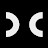Coding Cart says:

If you enjoyed this video, make sure to checkout my other video Capitalize! problem from HackerRank – https://www.youtube.com/watch?v=iV2ezsexlE8. Don't forget to like , subscribe and share with others who can learn from it. Thanks.

2.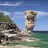Aryan Gupta says:

HELP!! why it s not working in opposite manner where arr be converted into set

3.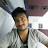phani raju says:

#import numpy as np

n,m=input().split()
k=[]

A=set()

B=set()

for i in range(int(n)):

s=input()

k.append(s)

for j in range(int(m)):

s=input()

A.add(s)

for j in range(int(m)):

s=input()

B.add(s)

#k=np.array(k)

k=list(k)

c=0

d=0

for i in k:

if i in A:

c+=1

elif i in B:

d+=1

else:

print(0)

print(c-d)

I gave this .but not coming

4.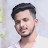sunny bisht says:

really helpful !!

5.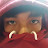Suthirak Prom says:

what do you use to draw on a macbook? I'm just curious.